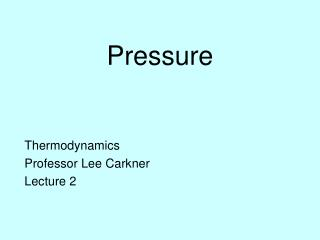# Pressure - PowerPoint PPT PresentationDownload PresentationPressure

PressureDownload Presentation## Pressure

- - - - - - - - - - - - - - - - - - - - - - - - - - - E N D - - - - - - - - - - - - - - - - - - - - - - - - - - -
##### Presentation Transcript

1. Pressure Thermodynamics Professor Lee Carkner Lecture 2

2. Pressure • Force per unit area • SI unit: Pascal • Pascals are really small so we often use kilopascals • Atmospheric pressure is about 100 kPa • More precisely: 1atm = 101325 Pa

3. Measuring Pressure • But, we mostly are dealing with things experiencing atmospheric air pressure • Pressures less than air pressure are vacuum pressures • We usually measure gage pressure but want absolute pressure

4. Pressure with Depth • The pressure of a column of fluid depends only on depth • This assumes constant density • For non-constant density the relationship is • We would need a expression for density as a function of depth (z) to solve

5. Multiple Fluids • Different fluids mixed together with different densities tend to settle out • The pressure at any point in the mixture is just the sum of all the rgh above that point

6. Barometer • Since P = rgh, we can measure pressure with a column of fluid of known density • Since air pressure is very large, we need a dense fluid to keep the device from being too large • Densest readily available fluid is mercury • One mm mercury = 1 torr = 133.3 Pa

7. Pascal’s Principle • Not only is pressure independent of the shape of a container, any pressure applied to a fluid is transmitted equally to all walls of the container • Known as Pascal’s Principle

8. Ideal Mechanical Advantage • Consider a curved pipe with ends of unequal width • If we press down with force F1 on the narrow end, the force on the wide end is: • A2/A1 is called the ideal mechanical advantage, and determines the amount by which our input force is multiplied

9. Manometer • Can evacuate the part of the tube above the fluid to measure absolute pressure, or keep it open to the air to measure gage pressure

10. Pressure Drops • The manometer will now measure the difference in pressure between the two points • The end with the greater pressure will push the manometer fluid down further • The difference in the height of the manometer fluid (h) is proportional to the pressure difference

11. Pressure Difference • The manometer fluid has pressure r2gh and the flow system fluid has pressure r1gh • The pressure difference is: • Can monitor fluid level to see if there is a problem with the flow

12. Pressure Measuring Devices • Manometers are often not practical • Want a pressure sensor that is easy to use and read • When fluid flows through the tube it tries to straighten out and moves the needle • Most convenient are electronic sensors

13. Next Time • Read: 2.2-2.5 • Homework: Chapter 2: 7, 28, 30